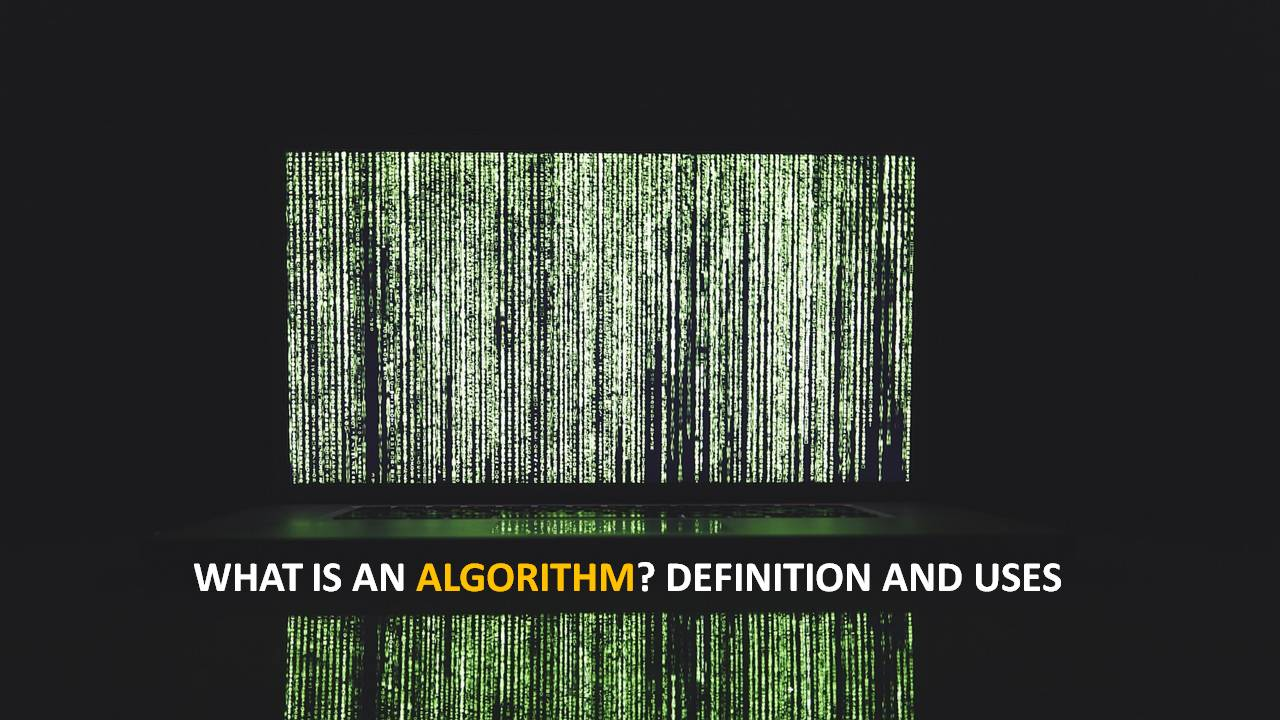23 Sep 2023

## Blog Post# What is an Algorithm? Definition and Uses

## Definition Algorithm

An algorithm is a finite group of operations organized in a logical manner that allows solving a particular problem. It is a series of instructions that, through a succession of steps, allow arriving at a result or solution.

Arabic is the etymological origin of the term algorithm that we are now going to analyze in-depth. More precisely, it is in the name of the mathematician Al-Khwarizmi. He was born in the Middle Ages in one of the areas of what is now known as Uzbekistan, in Central Asia.

It was in Baghdad that he developed a large part of his career and that he moved there too. By order of the caliph, he creates a superior center of scientific research called the House of Wisdom.

Different treatises of algebra or astronomy were some of the works of the said wise man. He has also given rise to the creation of another series of scientific terms such as algebra or figure.

Such was the importance of this historical figure that is currently considered as the father of algebra. He is also is known as the person who introduced our numbering system.

According to experts in mathematics, algorithms allow you to work from a basic or initial state after following the steps proposed, to reach a solution. It should be noted that, although algorithms are usually associated with the mathematical field (since they allow, to cite specific cases, find the quotient between a pair of digits or determine which is the greatest common divisor between two figures belonging to the group of integers), although they do not always imply the presence of numbers.

## Algorithm Uses

In addition to the above, in the mathematical field, and when we are determined to carry out the description of one of these algorithms, we must bear in mind that it can be done through three levels.

So, in the first place:

1. We find the high level, what is the formal description and finally the implementation task.
2. Algorithms can be expressed through programming languages, pseudo code, natural language, and also through those known as flow diagrams.
3. An instruction manual for the operation of an appliance and a series of orders from the boss to an employee to develop a certain task can also include algorithms.

This breadth of meaning allows us to appreciate that there is no formal and unique definition of an algorithm. The term is usually signaled as the fixed number of steps necessary to transform input information (a problem) into an output (its solution). Anyway, some algorithms have no end or do not solve a particular problem.

There are certain properties that reach all algorithms, with the exception of the so-called parallel algorithms.

• Sequential time (the algorithms work step by step).
• The abstract state (each algorithm is independent of its implementation).
• Bounded exploration (the transition between states is determined by a finite and fixed description).

Finally, it is worth mentioning that algorithms are very important in computing. Since they allow data to be represented as bit sequences. A program is an algorithm that tells the computer what specific steps to follow to develop a task.

Also Read: What is Bandwidth? Definition and Uses